# Hr to min

Sue biked to school in 5/12 of an hour. How many minutes did it take her to ride to school?

Result

m =  25 min

#### Solution:Leave us a comment of example and its solution (i.e. if it is still somewhat unclear...):

Showing 0 comments:Be the first to comment!## Next similar examples:

1. Speeds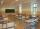George pass on the way to school distance 200 meters in 165 seconds. What is the average walking speed in m/s and km/h?
2. HoursThe lesson lasts 45 minutes. For the week, students have 18 lesson hours. How many are the actual hours?
3. Nightingale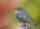Nightingale sang five seconds for 5 hours and 5 minutes. How many seconds were missing to sing 10 hours?
4. WorkersThree factory operators produced 480 units in 50 minutes. How many hours worked? I'm trying to prove or disapprove the idea that the company tell me that it's 2.5 hours. So what is right? Thank you, Petra
5. Clock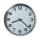How many hours are 15 days?
6. Time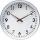16.2 days ..... how many hours is it?
7. Hours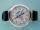How many hours is 9 days?
8. Bean bag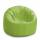A student tossed a bean bag. It landed 216 inches away. How many yards are equal to 216 inches?
9. Work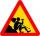Workers works 5 days a week and has 21 days of vacation a year. Calculate how many days is out of work and express it as a percentage. Consider that a year has 52 weeks.
10. Father and son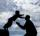Father is 44 years old, his son 16 years. Determine how many years ago was the father five times older than the son.
11. Plane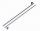On how many parts divide plane 6 parallels?
12. Foot in busIt was 102 people on the bus. 28 girls had two dogs. A 11 girls had one dog. At the next stop seceded 5 dogs (even with their owners). They got two boys together with three dogs. The bus drove one driver. How many foot were in bus?
13. Minutes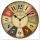Determine the difference in minutes: T1 = 2 3/20 h T2 = 2.3 h
14. Fan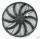The fan has a speed of 210 RPM. Calculate for time of one fan period.
15. Addition of Roman numbersAdded together and write as decimal number: LXVII + MLXIV
16. If-then equationIf 5x - 17 = -x + 7, then x =
17. Valid numberRound the 453874528 on 2 significant numbers.